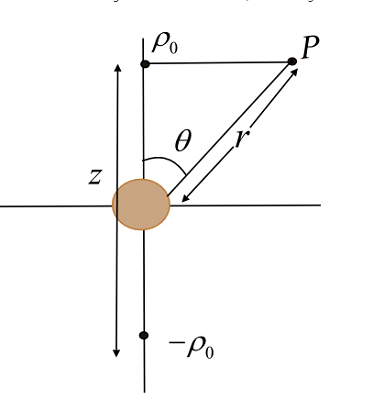×
Get Full Access to Introduction To Electrodynamics - 4 Edition - Chapter 3 - Problem 35p
Get Full Access to Introduction To Electrodynamics - 4 Edition - Chapter 3 - Problem 35p

×

A solid sphere, radius R, is centered at the origin. TheISBN: 9780321856562 45

Solution for problem 35P Chapter 3

Introduction to Electrodynamics | 4th Edition

• Textbook Solutions
• 2901 Step-by-step solutions solved by professors and subject experts
• Get 24/7 help from StudySoup virtual teaching assistantsIntroduction to Electrodynamics | 4th Edition

4 5 1 350 Reviews
25
2
Problem 35P

A solid sphere, radius R, is centered at the origin. The “northern” hemisphere carries a uniform charge density $$\rho_{0}$$, and the “southern” hemisphere a uniform charge density $$\rho_{0}$$. Find the approximate field E(r,θ) for points far from the sphere (r ≫ R).

.

.

Step-by-Step Solution:

Step 1 of 3

As the total charge for the full sphere is zero, we have to use the concept of a dipole. The diagram of the following problem can be shown as below:The electric field for a dipole at any distance is given by,The dipole moment is,Step 2 of 3

Step 3 of 3

ISBN: 9780321856562

Since the solution to 35P from 3 chapter was answered, more than 1280 students have viewed the full step-by-step answer. The answer to “?A solid sphere, radius R, is centered at the origin. The “northern” hemisphere carries a uniform charge density $$\rho_{0}$$, and the “southern” hemisphere a uniform charge density ?$$\rho_{0}$$. Find the approximate field E(r,?) for points far from the sphere (r ? R)...” is broken down into a number of easy to follow steps, and 42 words. This full solution covers the following key subjects: uniform, sphere, Density, Charge, hemisphere. This expansive textbook survival guide covers 12 chapters, and 550 solutions. The full step-by-step solution to problem: 35P from chapter: 3 was answered by , our top Physics solution expert on 07/18/17, 05:41AM. Introduction to Electrodynamics was written by and is associated to the ISBN: 9780321856562. This textbook survival guide was created for the textbook: Introduction to Electrodynamics , edition: 4.

Unlock Textbook Solution